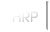Horse Racing Game Saturday, 8/17/2019 7:17 ET
 email
 password
 Handicapping System
 Below is an outline of how the weight assignments are calculated for Handicapping races. The following point system will be used to calculate points for each horse: - Grade I Stake - 1st=20, 2nd=8, 3rd=3 - Grade II Stake - 1st=15, 2nd=6, 3rd=2 - Grade III Stake - 1st=10, 2nd=4, 3rd=1 - Ungraded Stake - 1st=5, 2nd=2 - Everything else but Mdns, Clmrs, and Str Alws - 1st=1 - Maidens, Claimers, and Starter Allowances - 1st=0.5 * A horse will get half the points if it beats 1 horse and full points if it beats 2 or more horses. The points above will be multiplied by the below pecentages depending on when the win, place, or show took place. - Less than 2 months x 100% - Between 2-4 months x 90% - Between 4-6 months x 80% - Between 6-8 months x 70% - Between 8-10 months x 50% - Between 10-12 months x 25% The races will be categorized into one of the following 4 groups: less than 1 mile dirt race, 1 mile or more dirt race, less than 1 mile turf race, 1 mile or more turf race. The point system above will be used to calculate points for each of these categories for each horse and rounded to the nearest integer. The points for each category will be averaged. Not all races will count as a full race even though points are given when averaging. Take a look at the chart below. Grade I - 1st=0.2, 2+=1 Grade II - 1st=0.4, 2+=1 Grade III - 1st=0.6, 2+=1 Ungraded - 1st=0.8, 2+=1 Others - 1st=0, 2+=1 So, if a horse wins a GI, comes in 2nd in a GII, finished 3rd in an allowance, and finishes 3rd in a maiden all in the same category then it would receive 20 + 6 = 26 pts for the category. This would then be divided by the number of races which is equal to .2 + 1 + 1 + 1 = 3.2. The minimum number of races to divide by is 3. So the horse's rating would be 26 / 3.2 = 8.125. This number is then multiplied by 2.5 to get 20.3125 which would be rounded to 20. To get the final total for the category the below formula will be used: - (Matching Category + (Matching Surface Category x 33%) + (Matching Distance Category x 66%) + (Non Matching Category x 10%)) For example, say a given horse has the following points: 25 for DS, 10 for DL, 20 for TS, 5 for TL. The horse is entered in a DS Handicap so its point would be 25 + (10 * .33) + (20 * .66) + (5 * .10) = 42. Races for younger horses and females receive only a portion of the total points earned as well. Points earned for 3yo only races are multiplied by 0.85, points for 2yo only races are multiplied by 0.70, and points for female only races are multiplied by 0.85. The number of points for each horse is then plugged into the following formula: 130-((61-pts)/2) and rounded to the nearest integer. So, in the above example we would come up with 130-((61-42)/2)=121 and this would be the horse's rating for a dirt sprint. The final number for each horse is then taken and used in the final weight calculation below. - Grade I 126lbs maximum weight, 2pts=1lb - Grade II 127lbs maximum weight, 1.75pts=1lb - Grade III 128lbs maximum weight, 1.5pts=1lb - Ungraded 140lbs maximum weight, 1pt=1lb For Graded Handicaps the system starts by centering the weights around 119lbs. If the top horse carries more than the max then everything is shifted down so that the top horse does not carry more than the max weight. For Ungraded Handicaps the system also starts by centering the weights around 119lbs. If the bottom horse is less than 108lbs then the weight is shifted up until the bottom horse carries at least 108lbs or the top horse carries 140lbs. Here is an example. In a GI horse 1 has a rating of 130, horse 2 120, horse 3 114, and horse 4 100. The range is 30. Horse 1 will be assigned 119+(((130-100)-(30/2))/2)=127. Horse 2 will be assigned 119+(((120-100)-(30/2))/2)=122. Horse 3 will be 119+(((114-100)-(30/2))/2)=119. Horse 4 will be 119+(((100-100)-(30/2))/2)=112. Since the top horse is over 126 everyone will be shifted down 1 lb. The final weights would be 126,121,118,111. The weights for handicaps will be made available following the maintenance period after the nomination deadline. Handicap nomination deadlines will be 1 day earlier than other races. Owners will have until the next maintenance period after the nomination deadline to scratch out horses in handicaps for a reduced fee. After the next maintenace period the fee will go to the normal scratch fee. The entries, post positions, and jockey assignments will not be revealed until the fee is increased to the normal fee. Only weight will be available to the owner of each horse during the reduced fee period.
 You are connected to the unsecure web site. Click here to access the secure site. Copyright © 2003-2019 HorseRacingPark.com - horse racing game - virtual online realism, All Rights Reserved User Agreement  |  Privacy Policy  |  Sitemap